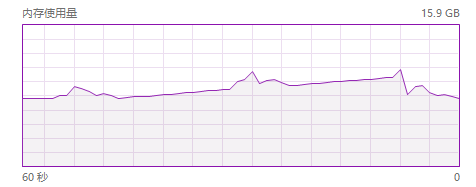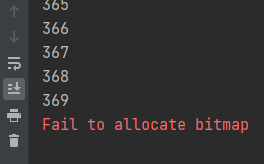# Methods to avoid fail to allocate bitmap errors in pyplot

1. Problem recurrence

After repeated drawing (mainly creating ` figure `), even if only the local variables inside the function are defined, or the drawing has been closed with
` PLT. Close() `, ` pyplot ` still retains some contents, but the specific part has not been found yet.

The following is a bug recurrence fragment from GitHub:

``````import matplotlib.pyplot as plt
import numpy as np
image = np.random.randn(256, 256, 3)
image= np.array(image/image.max()*255, dtype= np.uint8)
for i in range(500):
print(i)
plt.cla()
plt.imshow(image)
plt.close()
``````

After running, an error will be reported when ` I = 369 `, and the memory will continue to rise before the error is reported.memory usage change

error screenshot

2. Reason speculation

The drawing library based on ` Matplotlib ` may create window objects during drawing, but the related objects are not released when ` close() `, resulting in continuous memory accumulation.

3. Solutions

Define a ` figure ` and ` axes ` object and reuse it. Use ` cla() ` to clear the previous drawing content before each redrawing
example 1:

``````import matplotlib.pyplot as plt
import numpy as np
image = np.random.randn(256, 256, 3)
image= np.array(image/image.max()*255, dtype= np.uint8)
fig, axes= plt.subplots()
for i in range(500):
print(i)
axes.cla()
axes.imshow(image)
``````

Example 2:

``````import matplotlib.pyplot as plt
import numpy as np

class __:
def __init__(self):
self.fig, self.axes= plt.subplots()

def imshow(self, x):
self.axes.cla()
self.axes.imshow(image)

_= __()
image = np.random.randn(256, 256, 3)
image= np.array(image/image.max()*255, dtype= np.uint8)
for i in range(500):
print(i)
_.imshow(image)
``````